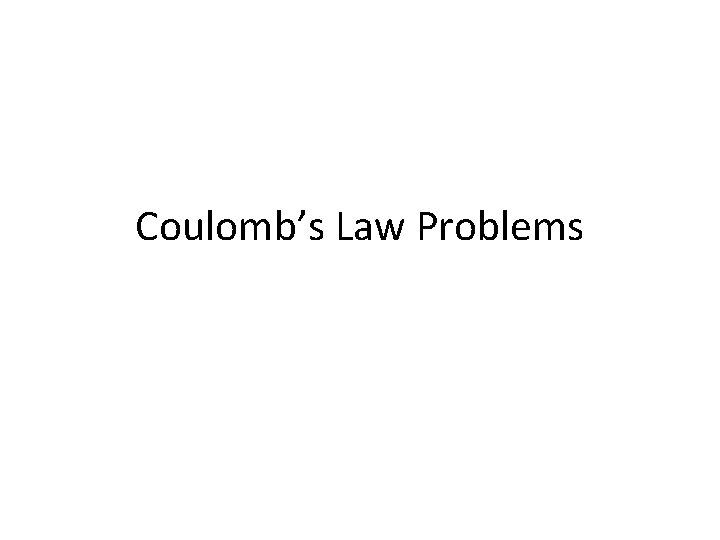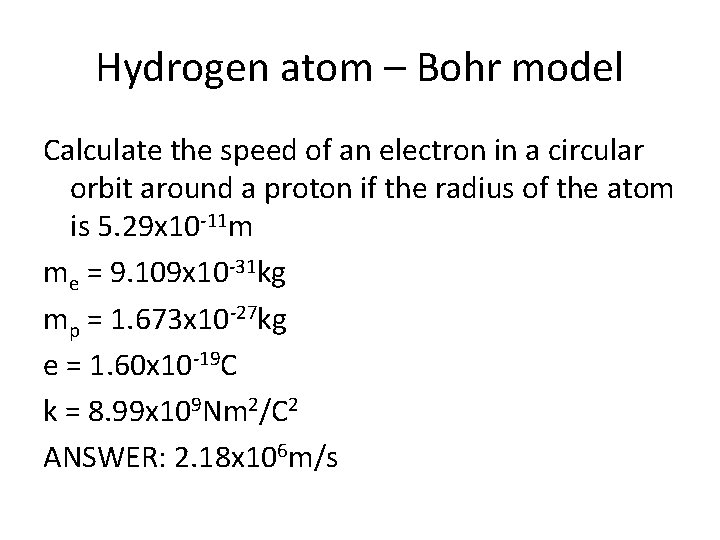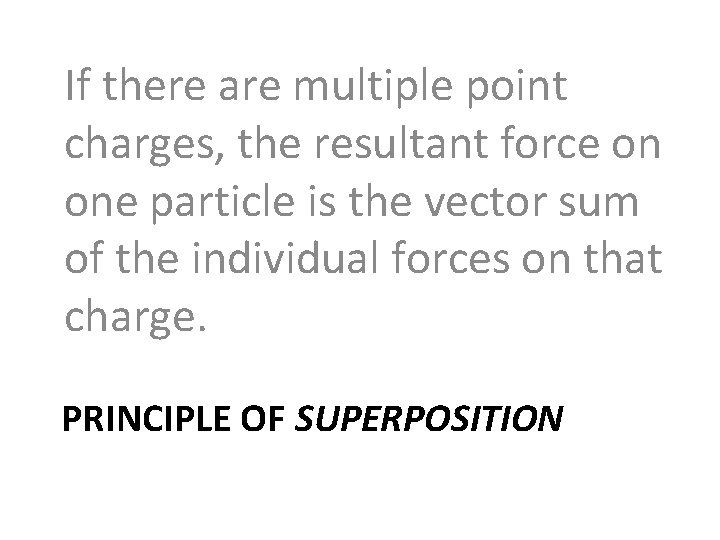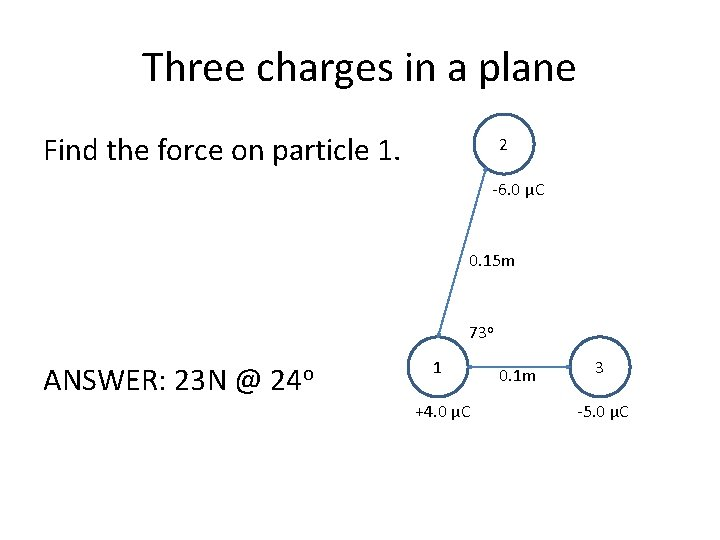# Coulombs Law Problems Gravity vs electric force The

• Slides: 8Coulomb’s Law ProblemsGravity vs. electric force The electron and proton of a hydrogen atom are separated, on average, by a distance of about 5. 3 x 10 -11 m. Find the magnitudes of the electric force and the gravitational force that each particle exerts on the other. me = 9. 109 x 10 -31 kg mp = 1. 673 x 10 -27 kg e = 1. 60 x 10 -19 C k = 8. 99 x 109 Nm 2/C 2 ANSWER: Fe = 8. 2 x 10 -8 N, Fg = 3. 6 x 10 -47 NLarge electric force Calculate the force between a +1 C and -1 C object if they are placed 1. 0 km away from each other. ANSWER: 9000 NHydrogen atom – Bohr model Calculate the speed of an electron in a circular orbit around a proton if the radius of the atom is 5. 29 x 10 -11 m me = 9. 109 x 10 -31 kg mp = 1. 673 x 10 -27 kg e = 1. 60 x 10 -19 C k = 8. 99 x 109 Nm 2/C 2 ANSWER: 2. 18 x 106 m/sIf there are multiple point charges, the resultant force on one particle is the vector sum of the individual forces on that charge. PRINCIPLE OF SUPERPOSITIONThree charges on a line Find the force on particle 1. 2 -4. 0μC ANSWER: +5. 7 N 0. 2 m 1 +3. 0 μC 0. 15 m 3 -7. 0 μCThree charges in a plane Find the force on particle 1. 2 -6. 0 μC 0. 15 m 73 o ANSWER: 23 N @ 24 o 1 +4. 0 μC 0. 1 m 3 -5. 0 μCTwo 1. 1 g balloons are suspended from 2. 0 m long strings and hung from the ceiling. They are then rubbed 10 times with animal fur to impart an identical charge Q to each balloon. The balloons repel each other and each string is observed to make an angle of 15° with the vertical. Determine the electric force of repulsion, the charge on each balloon, and the quantity of electrons transferred to each balloon as a result of 10 rubs with animal fur.